Three points

Mark three points E, F and G in the plane not lie on one line.

a) Draw a line segment FG
b) Construct halfline (ray) EG
c) Draw a line EF

Leave us a comment of example and its solution (i.e. if it is still somewhat unclear...):Be the first to comment!Next similar examples:

1. Straight lines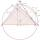Draw two lines c, d so that c || d. On line c mark points A, B, from point A start perpendicular to line c, from point B perpendicular to line c.
2. Draw it!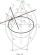Draw two lines c, d that c || d. On line c mark the points A, B. By point A lead perpendicular line to c. By point B lead perpendicular line to c.
3. Line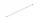How many parts of line divide 5 (different) points that lie on it?
4. Square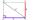Draw a square on the edge of a = 4 cm. Mark the center of symmetry S and all axes of symmetry. How many axes of symmetry does? Write down.
5. Plane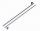On how many parts divide plane 6 parallels?
6. LineCan we construct a line segment, if we know: center
7. Train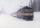The train passes part of the line for 95 minutes at speed 75 km/h. What speed would have to go in order to shorten the driving time of 20 minutes?
8. The sum graphically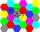Draw a graphically sum of the all sides of 4-gon ABCD.
9. TriangleProve whether you can construct a triangle ABC, if a=9 cm, b=10 cm, c=4 cm.
10. Roman numerals 2+Add up the number writtens in Roman numerals. Write the results as a roman numbers.
11. DoctorsIn the city operates 171 doctors. The city has 128934 citizens. How many citizens are per one doctor?
12. To thousands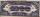Round to thousands following numbers:
13. Roman numeralsWrite numbers written in Roman numerals as decimal.
14. Roman numerals +Add up the number writtens in Roman numerals. Write the results as a decimal number.
15. Compare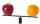Compare with characters >, <, =: 85.57 ? 80.83Added together and write as decimal number: LXVII + MLXIVResult of the product of the numbers 1, 2, 3, 1, 2, 0 is: